# problem 3 SEL 3. Prove that the mapping w 4. Prove that w z3 3z 1...

problem 3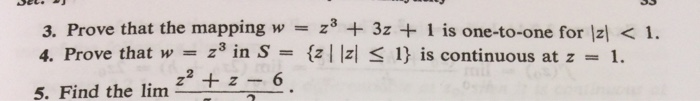SEL 3. Prove that the mapping w 4. Prove that w z3 3z 1 is one-to-one for |z <1 Z n S {z| |z < 1} is continuous at z 1 +z6 5. Find the lim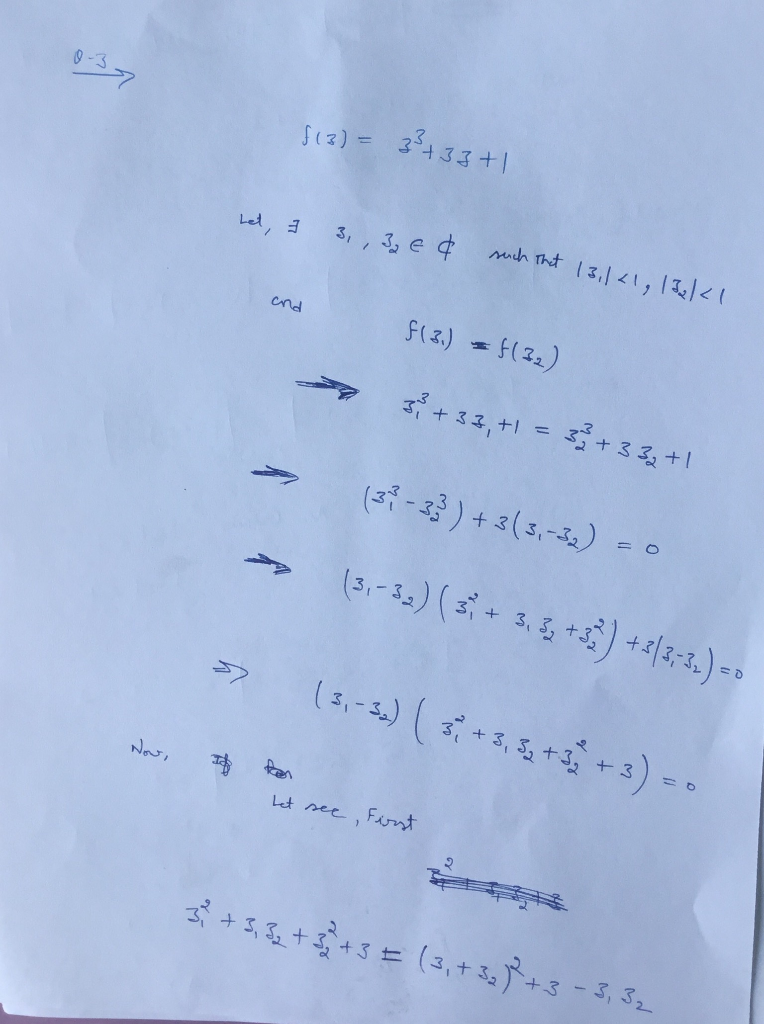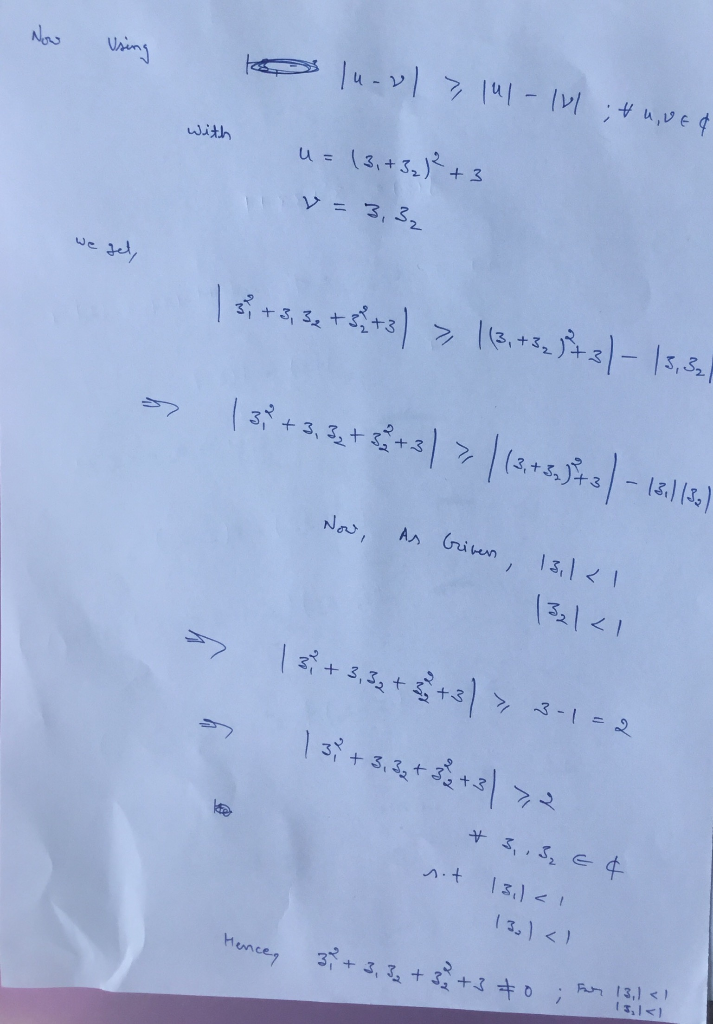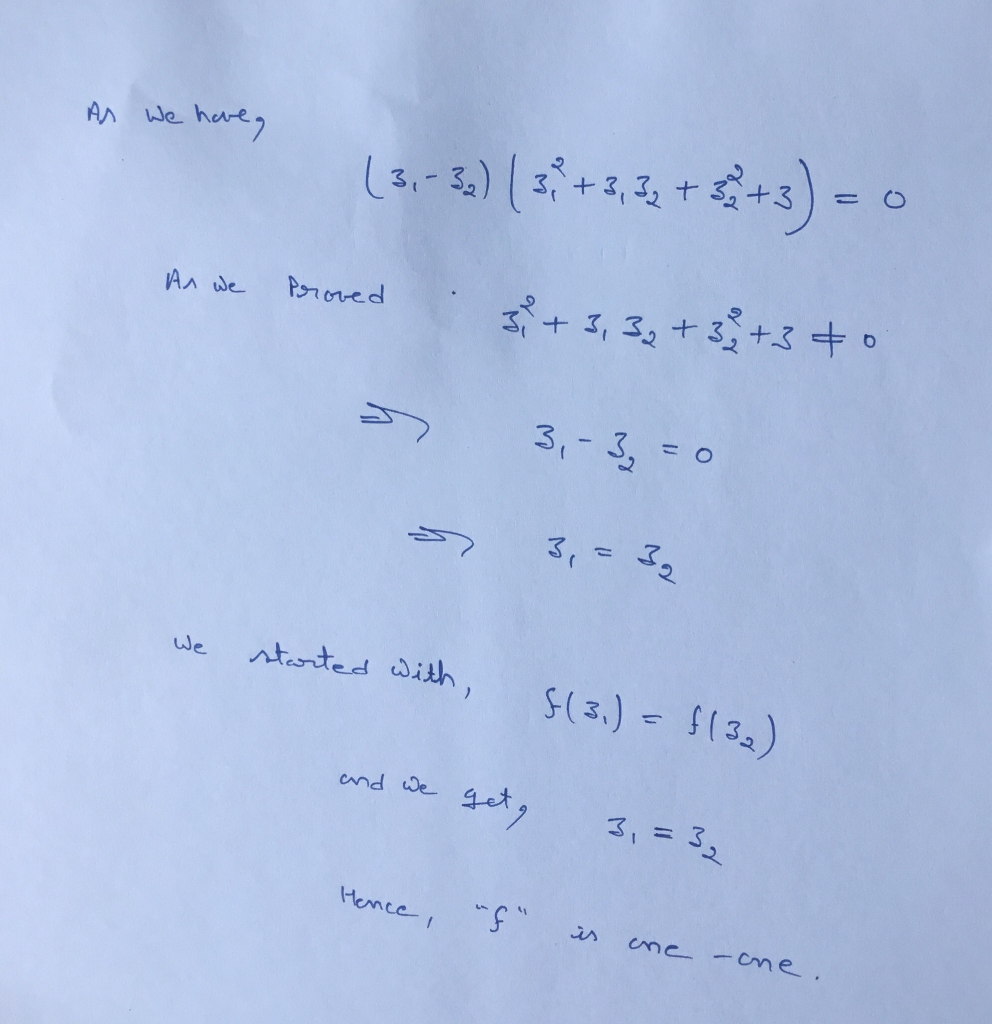#### Earn Coin

Coins can be redeemed for fabulous gifts.

Similar Homework Help Questions
• ### IDY in < oo and lim - Yn < 0o. Prove that lim,+ 1. Let In...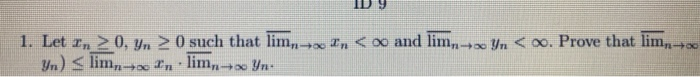IDY in < oo and lim - Yn < 0o. Prove that lim,+ 1. Let In > 0. Yn > 0 such that lim,- Yn) < lim,-- In lim,+ Yn: i tn < oo and lim yn < . Prove that lim. In 1. Let In 20, yn 0 such that lim Yn) < limn+In lim + Yr

• ### Let U ? Rmxn. Prove that if UTI-In, then n < m.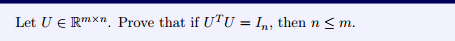Let U ? Rmxn. Prove that if UTI-In, then n < m.

• ### Let ne Nj. Prove that n < 2(6(n)).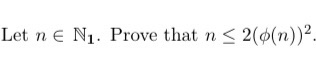Let ne Nj. Prove that n < 2(6(n)).

• ### 5. Prove that f(z) = (2+1/2) is a conformal map from the half-disc {z = x...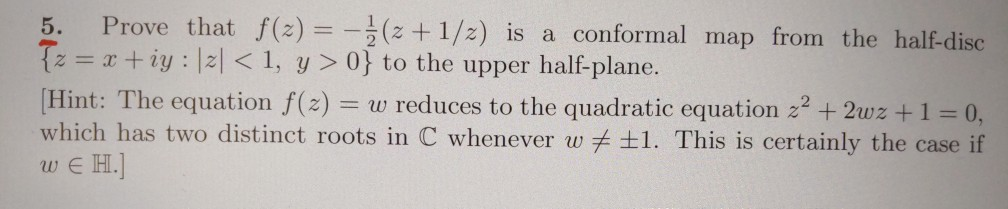5. Prove that f(z) = (2+1/2) is a conformal map from the half-disc {z = x +iy : 2< 1, y >0} to the upper half-plane. (Hint: The equation f(z) = w reduces to the quadratic equation z2 + 2wz +1 = 0, which has two distinct roots in C whenever w # £1. This is certainly the case if WE H.

• ### , then n lim Let Ά be a square matrix. Prove that if ρ(A)<1 Use the...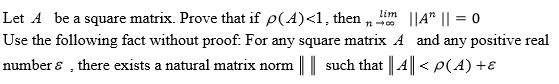, then n lim Let Ά be a square matrix. Prove that if ρ(A)<1 Use the following fact without proof. For any square matrix A and any positive real number ε , there exists a natural matrix norm I l such that l-4 ll < ρ (d) +ε IIA" 11-0

• ### 4. Prove that the external measure is not "additive", in the sense that [0, 1]le <...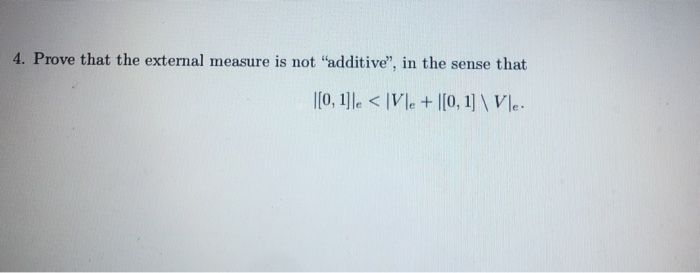4. Prove that the external measure is not "additive", in the sense that [0, 1]le < Vle+ |[0, 1] \Vle. 5. Let 7(x) := lim sup fx(x). +00 Prove that, for every a € R, {7 > a} = UN U{n>a + m}: MENJEN

• ### Prove using mathematical induction that 3" + 4" < 5" for all n > 2.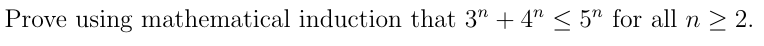Prove using mathematical induction that 3" + 4" < 5" for all n > 2.

• ### 3) Prove that there exists f : R → R non-negative and continuous such that f...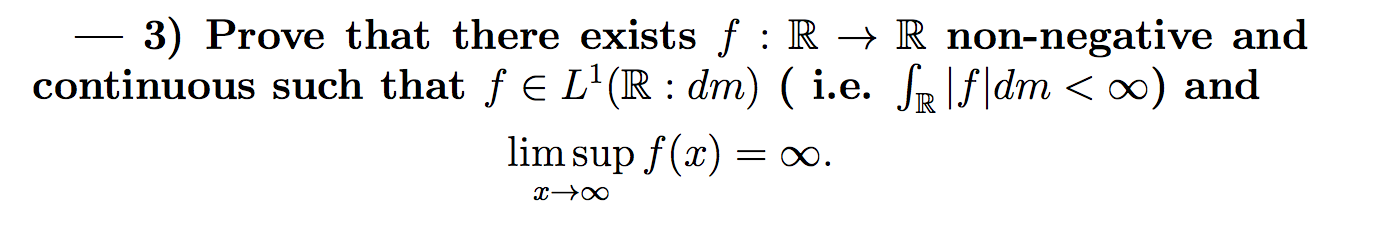3) Prove that there exists f : R → R non-negative and continuous such that f € L'OR : dm) ( i.e. SR \f|dm <00) and lim sup f(x) = ∞. 2-0

• ### 6. (20 pts) Prove that there is no homeomorphism of the closed interval (-1,1] of the...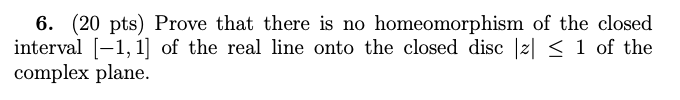6. (20 pts) Prove that there is no homeomorphism of the closed interval (-1,1] of the real line onto the closed disc < 1 of the complex plane.

• ### For each . Find the intersection of and prove. Please show and explain steps. neN. An...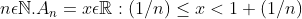For each . Find the intersection of and prove. Please show and explain steps. neN. An = zeR: (1/n) <<<1+(1/n) We were unable to transcribe this image